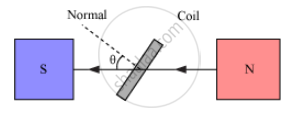Department of Pre-University Education, KarnatakaPUC Karnataka Science Class 12

# Obtain the expression for the emf induced in the rotating coil of N turns each of cross-sectional area A, in the presence of a magnetic field B - Physics

Obtain the expression for the emf induced in the rotating coil of N turns each of cross-sectional area A, in the presence of a magnetic field vecB .

#### Solution

As the armature coil is rotated in the magnetic field, the angle θ between the field and normal to the coil changes continuously. Therefore, magnetic flux linked with the coil changes. An emf is induced in the coil. According to Fleming’s right-hand rule, current induced in AB is from A to B and it is from C to D in CD. In the external circuit, current flows from B2 to B1.

To calculate the magnitude of emf induced:

Suppose

A → Area of each turn of the coil

N → Number of turns in the coil

vecB→ Strength of magnetic field

θ → Angle which normal to the coil makes with vecB at any instant t∴ Magnetic flux linked with the coil in this position:

phi=N(vecB.vecA)=NBA cos theta=NBA cos omega t     .....(I)

Where ‘ω’ is the angular velocity of the coil

As the coil rotates, angle θ changes. Therefore, magnetic flux Φ linked with the coil changes, and hence, an emf is induced in the coil. At this instant t, if e is the emf induced in the coil, then

e=-(d theta)/dt=-d/dt(NAB cos omega t)

=-NAB d/dt(cos omega t)

=-NAB(-sin omega t)omega

therefore = NAB omega sin omegat

Concept: AC Generator
Is there an error in this question or solution?